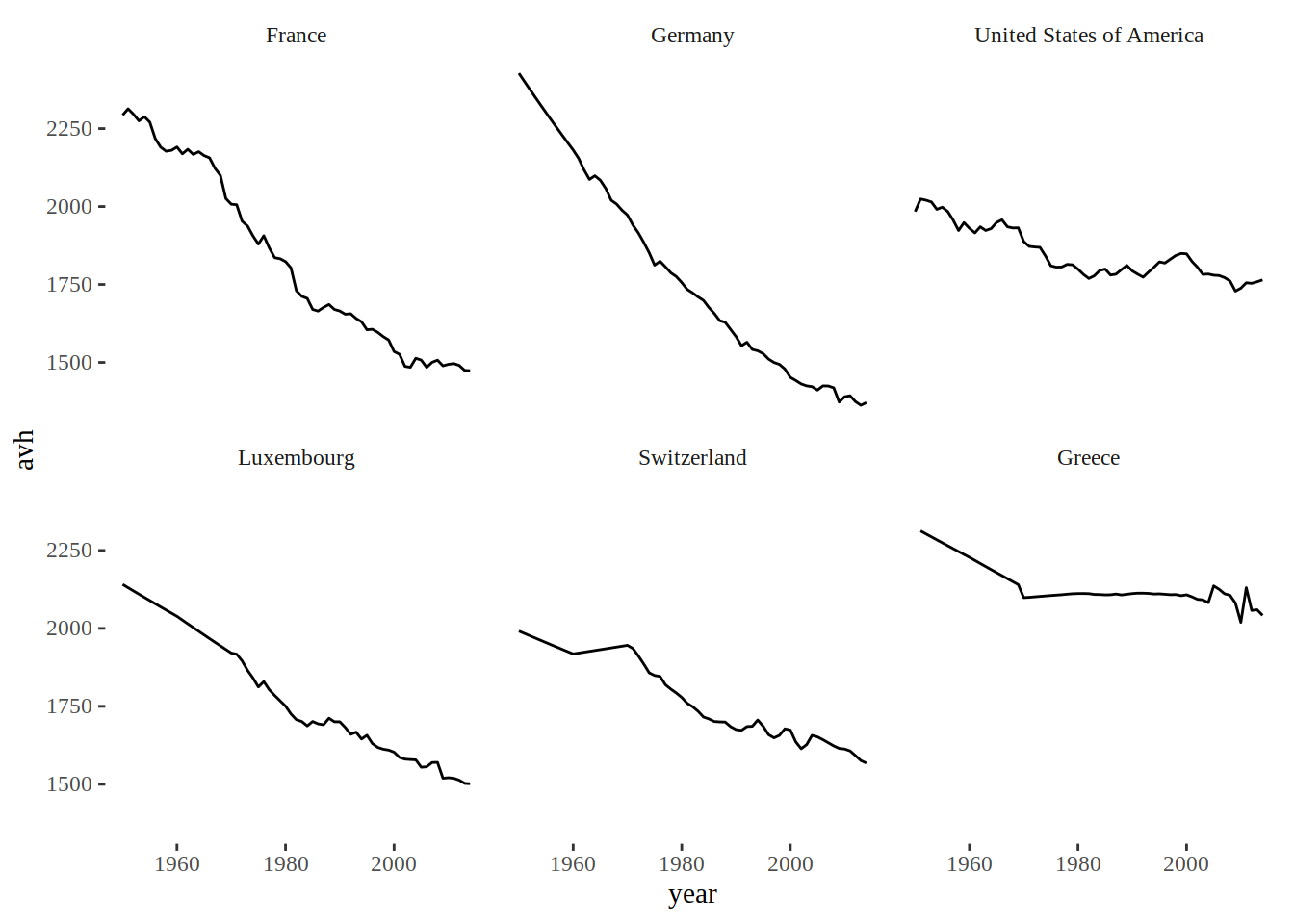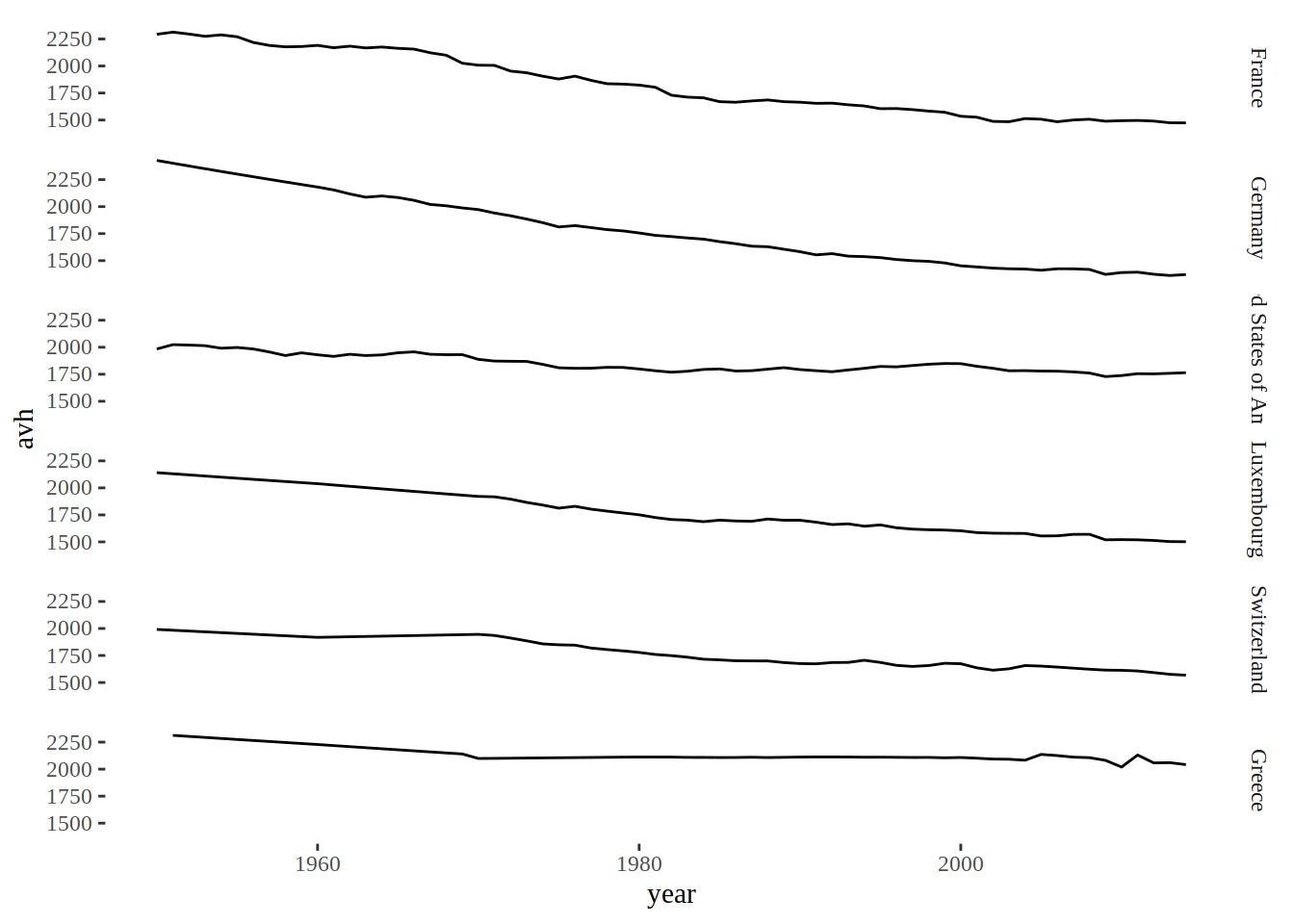```Econometrics and Free Software by Bruno Rodrigues.
Check out my package that adds logging to R functions, {chronicler}.
Or read my free ebooks, to learn some R and build reproducible analytical pipelines..
You can also watch my youtube channel or find the slides to the talks I've given here.
Buy me a coffee, my kids don't let me sleep.
```

# Make ggplot2 purrr

R

Update: I’ve included another way of saving a separate plot by group in this article, as pointed out by `@monitus`. Actually, this is the preferred solution; using `dplyr::do()` is deprecated, according to Hadley Wickham himself.

I’ll be honest: the title is a bit misleading. I will not use `purrr` that much in this blog post. Actually, I will use one single `purrr` function, at the very end. I use `dplyr` much more. However Make ggplot2 purrr sounds better than Make ggplot dplyr or whatever the verb for `dplyr` would be.

Also, this blog post was inspired by a stackoverflow question and in particular one of the answers. So I don’t bring anything new to the table, but I found this stackoverflow answer so useful and so underrated (only 16 upvotes as I’m writing this!) that I wanted to write something about it.

Basically the idea of this blog post is to show how to create graphs using `ggplot2`, but by grouping by a factor variable beforehand. To illustrate this idea, let’s use the data from the Penn World Tables 9.0. The easiest way to get this data is to install the package called `pwt9` with:

``install.packages("pwt9")``

and then load the data with:

``data("pwt9.0")``

Now, let’s load the needed packages. I am also using `ggthemes` which makes themeing your ggplots very easy. I’ll be making Tufte-style plots.

``````library(ggplot2)
library(ggthemes)
library(dplyr)
library(tidyr)
library(purrr)
library(pwt9)``````

First let’s select a list of countries:

``````country_list <- c("France", "Germany", "United States of America", "Luxembourg", "Switzerland", "Greece")

small_pwt <- pwt9.0 %>%
filter(country %in% country_list)``````

Let’s us also order the countries in the data frame as I have written them in `country_list`:

``````small_pwt <- small_pwt %>%
mutate(country = factor(country, levels = country_list, ordered = TRUE))``````

You might be wondering why this is important. At the end of the article, we are going to save the plots to disk. If we do not re-order the countries inside the data frame as in `country_list`, the name of the files will not correspond to the correct plots!

Update: While this can still be interesting to know, especially if you want to order the bars of a barplot made with `ggplot2`, I included a suggestion by `@expersso` that does not require your data to be ordered!

Now when you want to plot the same variable by countries, say `avh` (Average annual hours worked by persons engaged), the usual way to do this is with one of `facet_wrap()` or `facet_grid()`:

``````ggplot(data = small_pwt) + theme_tufte() +
geom_line(aes(y = avh, x = year)) +
facet_wrap(~country)````````````ggplot(data = small_pwt) + theme_tufte() +
geom_line(aes(y = avh, x = year)) +
facet_grid(country~.)``````As you can see, for this particular example, `facet_grid()` is not very useful, but do notice its argument, `country~.`, which is different from `facet_wrap()`’s argument. This way, I get the graphs stacked horizontally. If I had used `facet_grid(~country)` the graphs would be side by side and completely unreadable.

Now, let’s go to the meat of this post: what if you would like to have one single graph for each country? You’d probably think of using `dplyr::group_by()` to form the groups and then the graphs. This is the way to go, but you also have to use `dplyr::do()`. This is because as far as I understand, `ggplot2` is not `dplyr`-aware, and using an arbitrary function with groups is only possible with `dplyr::do()`.

Update: As explained in the intro above, I also added the solution that uses `tidyr::nest()`:

``````# Ancient, deprecated way of doing this
plots <- small_pwt %>%
group_by(country) %>%
do(plot = ggplot(data = .) + theme_tufte() +
geom_line(aes(y = avh, x = year)) +
ggtitle(unique(.\$country)) +
ylab("Year") +
xlab("Average annual hours worked by persons engaged"))``````

And this is the approach that uses `tidyr::nest()`:

``````# Preferred approach
plots <- small_pwt %>%
group_by(country) %>%
nest() %>%
mutate(plot = map2(data, country, ~ggplot(data = .x) + theme_tufte() +
geom_line(aes(y = avh, x = year)) +
ggtitle(.y) +
ylab("Year") +
xlab("Average annual hours worked by persons engaged")))``````

If you know `dplyr` at least a little bit, the above lines should be easy for you to understand. But notice how we get the title of the graphs, with `ggtitle(unique(.\$country))`, which was actually the point of the stackoverflow question.

Update: The modern version uses `tidyr::nest()`. Its documentation tells us:

There are many possible ways one could choose to nest columns inside a data frame. `nest()` creates a list of data frames containing all the nested variables: this seems to be the most useful form in practice. Let’s take a closer look at what it does exactly:

``````small_pwt %>%
group_by(country) %>%
nest() %>%
``````## # A tibble: 6 x 2
##   country                  data
##   <ord>                    <list>
## 1 Switzerland              <tibble [65 × 46]>
## 2 Germany                  <tibble [65 × 46]>
## 3 France                   <tibble [65 × 46]>
## 4 Greece                   <tibble [65 × 46]>
## 5 Luxembourg               <tibble [65 × 46]>
## 6 United States of America <tibble [65 × 46]>``````

This is why I love lists in R; we get a `tibble` where each element of the column `data` is itself a `tibble`. We can now apply any function that we know works on lists.

What might be surprising though, is the object that is created by this code. Let’s take a look at `plots`:

``print(plots)``
``````## # A tibble: 6 x 3
##   country                  data               plot
##   <ord>                    <list>             <list>
## 1 Switzerland              <tibble [65 × 46]> <S3: gg>
## 2 Germany                  <tibble [65 × 46]> <S3: gg>
## 3 France                   <tibble [65 × 46]> <S3: gg>
## 4 Greece                   <tibble [65 × 46]> <S3: gg>
## 5 Luxembourg               <tibble [65 × 46]> <S3: gg>
## 6 United States of America <tibble [65 × 46]> <S3: gg>``````

As `dplyr::do()`’s documentation tells us, the return values get stored inside a list. And this is exactly what we get back; a list of plots! Lists are a very flexible and useful class, and you cannot spell list without `purrr` (at least not when you’re a ne`R`d).

Here are the final lines that use `purrr::map2()` to save all these plots at once inside your working directory:

Update: I have changed the code below which does not require your data frame to be ordered according to the variable `country_list`.

``````# file_names <- paste0(country_list, ".pdf")

map2(paste0(plots\$country, ".pdf"), plots\$plot, ggsave)``````

As I said before, if you do not re-order the countries inside the data frame, the names of the files and the plots will not match. Try running all the code without re-ordering, you’ll see!